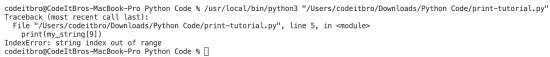# How to Handle String Index Out of Range Error In Python

In this tutorial, we will discuss how to handle string index out of range error. Before moving ahead, let’s first understand what is string index out of range error is in Python.

Just like lists, strings are also indexed in Python. We can define a string as a set of Unicode characters stored inside. In simpler terms, you can access each item in a string using its index value which starts with zero. So the first character index is 0, and the last character index is equal to the length of the string-1.

Whenever you try to access an item whose index value does not exist in the string, an error is thrown by the Python interpreter known as string index out of range.

Also ReadPython For Loop Index With Examples

## What is String Index Out of Range Error In Python

Let us consider a string: Codeitbro. In this string, the index of C is 0, and the index of o is 8 (as the length of the string is 9).

When accessing the characters inside a string, we can use their index values to access them. However, if you use an index not available in the string, you will get the index out of range error.

As in the above example, the index range is only up to 8. but if we try to access it using an index value of 10, we will get the error.

Let’s see the error in the below code to get a value at the 10th index position.

```#consider a string
my_string = "Codeitbro"

#get the tenth charcater
print(my_string)
```

Error:```IndexError                                Traceback (most recent call last)
<ipython-input-22-5d6870b88bcc> in <module>()
3
4 #get the tenth charcater
----> 5 print( my_string)
6

IndexError: string index out of range```

## How to Handle String Index Out of Range Error In Python

To handle this error, we will see the following methods.

### Method 1: Use the relevant index

We have to use the index of a character in the string only. To get the character by indexing from the string, you can use [].

Syntax:

`my_string[index]`

Where,

1. my_string is the input string.

2. index is the character index in the string that is present.

Example:

We will create a string and get the character using an index in this example.

```#consider a string
my_string = "Codeitbro"

#get the first charcater
print( my_string)

#get the fifth charcater
print( my_string)

#get the second charcater
print( my_string)

#get the last charcater
print( my_string)
```

Output:

```C
i
e
o```

### Method 2: Using Exception Handling to Handle String Index Out of Range Error

We will use the exception handling concept to handle the error in this scenario. For this, we will use try and except code blocks.

Here’s the syntax:

```try:
statements

except IndexError:
handling```

In the try block, we will use statements to retrieve a value based on an index value in the string. If there is no error, it will return the character. Otherwise, Python will execute the statements in the except block to handle the error.

Let’s see a few examples to understand this more coherently.

Example 1: In this example, we will create a string and try to get the tenth character using index and display an error message if there is any string index out of range error under the except block.

Code:

```#consider a string
my_string = "Codeitbro"

try:
#get the tenth charcater
print( my_string)

except IndexError:
print("Index error occured")

```

Output:

`Index error occured`

Example 2: We will create a string and get the seventh character using the index in this example.

```#consider a string
my_string = "Codeitbro"

try:
#get the seventh charcater
print( my_string)

except IndexError:
print("Index error occured")

```

There is no error found in the try block of the above code, and therefore it prints the sixth character of the string, i.e., “b.”

Output:

`b`

### Wrapping Up

In this tutorial, we explored two methods to handle string index out of range error in Python. The first is pretty straightforward, in which you have to use the correct index to get a value from the string. The second method is robust as it uses the exception handling concept to handle the index out of range error.